# HCTF 2018 Writeup

HCTF 2018 had been held for 48 hours from November 9th. HCTF is held every year but this was the first time for us to participate in HCTF. I joined in the CTF as a member of team insecure. We got 3032.49pt and I solved 4 challenges.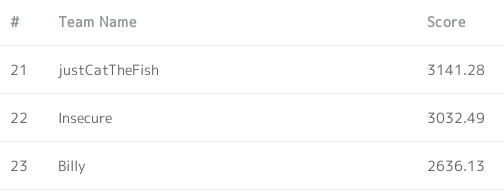Thank you for the awsome CTF. I learned a lot!

# [Web] Warmup

Description:
warmup
URL: http://warmup.2018.hctf.io
Team solved: 266

There are index.php, hint.php on the given url and we can also access to source.php, which I found in the source code of index.php. The source.php tells us the whole PHP code of index.php.

 <?php
class emmm
{
public static function checkFile(&$page) {$whitelist = ["source"=>"source.php","hint"=>"hint.php"];
if (! isset($page) || !is_string($page)) {
echo "you can't see it";
return false;
}

if (in_array($page,$whitelist)) {
return true;
}

$_page = mb_substr($page,
0,
mb_strpos($page . '?', '?') ); if (in_array($_page, $whitelist)) { return true; }$_page = urldecode($page);$_page = mb_substr(
$_page, 0, mb_strpos($_page . '?', '?')
);
if (in_array($_page,$whitelist)) {
return true;
}
echo "you can't see it";
return false;
}
}

if (! empty($_REQUEST['file']) && is_string($_REQUEST['file'])
&& emmm::checkFile($_REQUEST['file']) ) { include$_REQUEST['file'];
exit;
} else {
echo "<br><img src=\"https://i.loli.net/2018/11/01/5bdb0d93dc794.jpg\" />";
}
?>


It's a whitelist check to verify the file parameter is "source.php" or "hint.php". The hint says that the filename of the flag is ffffllllaaaagggg. As we can see in the code, only the token before '?' will be checked. For example, parameters like file=source.php?hello will pass the filter. However it won't include any files because there are no such files named source.php?hello. After several attempts, I realized that I could bypass the filter by using a relative path like:

http://warmup.2018.hctf.io/?file=hint.php?/../hint.php

Finally I found the flag in the root directory.

http://warmup.2018.hctf.io/?file=hint.php?/../../../../ffffllllaaaagggg

# [Misc] freq game

Description:
this is a eazy game. nc 150.109.119.46 6775
Team solved: 36

We can get the source code by entering 'hint.'

$nc 150.109.119.46 6775 _______ _______ _______ _______ _______ _______ _______ _______ ( ____ \( ____ )( ____ \( ___ ) ( ____ \( ___ )( )( ____ \ | ( \/| ( )|| ( \/| ( ) | | ( \/| ( ) || () () || ( \/ | (__ | (____)|| (__ | | | | | | | (___) || || || || (__ | __) | __)| __) | | | | | | ____ | ___ || |(_)| || __) | ( | (\ ( | ( | | /\| | | | \_ )| ( ) || | | || ( | ) | ) \ \__| (____/\| (_\ \ | | (___) || ) ( || ) ( || (____/\ |/ |/ \__/(_______/(____\/_) (_______)|/ \||/ \|(_______/ this is a sample game ... input y to start this game, and input hint to get hint: We are given the following python code which is running on the server. #!/usr/bin/env python def get_number(x, freq,rge): y = np.sin(2*np.pi*x*freq)*rge return y def divide_flag(token): flag_list = [] flag = "****************************************************************" for i in range(0,64,2): flag_list.append(int(flag[i]+flag[i+1],16)) return flag,flag_list def game(level,flag_list): level = level*4 freq_list = flag_list[level:level+4] x = np.linspace(0,1,1500) y = [] for freq in freq_list: if y == []: y = get_number(x,freq,7) else: y += get_number(x,freq,7) return y,freq_list def start_game(tcpClisock,token,userlogger): flag,flag_list = divide_flag(token) level = 0 while True: if level == 8: tcpClisock.sendall((CONGRATULATION_TXT.format("hctf{"+flag+"}")).encode("utf-8")) break y,freq_list = game(level,flag_list) send_data = json.dumps(list(y)).encode("utf-8") tcpClisock.sendall(send_data) req_data = tcpClisock.recv(1024).decode("utf-8").replace("\n","") req = req_data.split(" ") req.sort() freq_list.sort() if req == freq_list: level += 1 continue else: break tcpClisock.close()  We have to pass 8 rounds and 4 different frequencies are used in every round. Let the frequencies be, then the data we get is:What we have to send is a list of the frequenciesto. We can retrieve every frequencies by applying Fourier transform. from ptrlib import * import json import numpy as np token = "*** Every team has a token ***" sock = Socket("150.109.119.46", 6775) sock.settimeout(1.0) sock.recvuntil("hint:") sock.sendline("y") sock.recvuntil("token:") sock.sendline(token) for r in range(8): print("Round {0}...".format(r + 1)) array_json = sock.recvall() y = json.loads(array_json) # FFT F = np.fft.fft(y) Amp = np.abs(F) freq = np.linspace(0.0, 0xFFFF, 1500) # Find freq freq_list = [] for c in xrange(0x100): if Amp[c] > 5000: freq_list.append(c) if len(freq_list) != 4: print("Error!") print(freq_list) exit(1) # Send answer data = "" for freq in freq_list: data += str(freq) + " " sock.sendline(data.rstrip()) print(sock.recvall()) sock.close()  By running this program, I could successfully get the flag. (Sorry for using a private CTF library.) # [Crypto] xor game Description: This is an English poem, but it is encrypted. Find the flag and restore it (also you can just submit the flag). URL: http://img.tan90.me/xor_game.zip Team solved: 98 The encryption algorithm is to xor the text repeatedly with the flag. Since the original text is (supposed to be) an English text, we can perform Kasiski examination and analyse the frequency of characters. The length of the poem seems large enough to break the cipher, so let's try it. We have to find the key length first. The following program finds the most possible key length with Kasiski examination. # Read the ciphertext as a list of ascii codes text = open("cipher.txt", "r").read().decode("base64") encoded = map(ord, list(text)) eq = lambda x: x == x # Try several key lengths max_freq = 0 guess_keylen = 0 for length in range(1, 50): shifted = encoded[length:] + encoded[:length] freq = map(eq, zip(encoded, shifted)).count(True) if max_freq < freq: max_freq = freq guess_keylen = length print("Key Length: {0}".format(guess_keylen))  It says the key length may be 21. Second, we have to find the key based on the key length and the frequency of letters. The challenge description says that the original text is an English poem. An english text must contain a lot of whitespaces. When we collect characters every 21 bytes in the ciphertext, the most frequently appeared character possibly corresponds to a whitespace. I used this technique and wrote a decoder. import string def dec(encrypted, key): key = (key * (len(encrypted) / len(key) + 1))[:len(encrypted)] xor = lambda x: x ^ ord(x) return map(xor, zip(encrypted, list(key))) def calc_freq(encrypted, keylen, i): # Calculate the frequency freq = {} for c in encrypted[i::keylen]: if c in freq: freq[c] += 1 else: freq[c] = 1 return freq def find_key(encrypted, keylen, key='', i=0): # Generate possible keys if len(key) == keylen: decoded = dec(encrypted, key) ascii_count = 0 for c in decoded: if chr(c) in string.printable: ascii_count += 1 if float(ascii_count) / len(encrypted) > 0.9: yield key else: freq = calc_freq(encrypted, keylen, i) # Get 3 common letters common = sorted(freq.items(), key=lambda x:-x)[:2] for candidate in common: k = candidate ^ 0x20 if chr(k) not in string.printable: # The key is not printable continue # Next character... for x in find_key(encrypted, keylen, key + chr(k), i + 1): yield x with open("cipher.txt", "rb") as f: binary = f.read().decode("base64") encrypted = map(ord, list(binary)) key = next(find_key(encrypted, 21)) print key print("===== Encrypted =====") print(repr(binary)) print("===== Decrypted =====") print repr("".join(map(chr, dec(encrypted, key))))  This decoder unfortunately doesn't find the correct key. However, it left me an important clue. I found the following piece in the decrypted text. ... es1in"\nRepea: *ut7i ... I realized that "\nRepea:" should be "\nRpeat" originally. Since this token appears at 162th, the 16th(162 % 21 = 15) to 21th letters of the key are correct and the 1st letter is wrong. The correct one should be: chr(ord("6")^ord(":")^ord("t")) = 'x' I fixed the 1st letter and tried to decrypt. Then I found the following piece. ... e agai+\n ... Yes, "agai+" should be "again", which means the 3rd(44 % 21 = 2) letter of the key is wrong. The correct one should be: chr(ord("7")^ord("+")^ord("n")) = 'r' In this way, I could successfully restore the flag: hctf{xor_is_interesting!@#}. # [Rev] LuckyStar☆ Description: Lucky channel! URL: https://mega.nz/#!6m4kHCIA!HaNLmfENJJ0Z_TKsX8Q7rWKJblP-m3dIjgcDYH-QYSk Team solved: 24 The file is an executable for Windows x86, named LuckyStar.exe. The program plays a Japanese anime song and we can input a flag and check if it's correct after the song ends. So, let's analyse it with x64dbg. At first, the program checks if some debuggers presents. However, it seemed to do nothing even though I had been running x64dbg. Anyway, after that it calls srand with a constant value 0x61616161 and unpacks a region using xor with rand().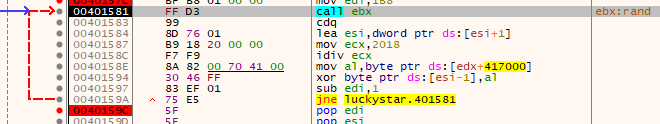This is just a trick to prevent a static analysis. The unpacked code is located at 0x00401780 and its the main routine. Let's set a breakpoint there and continue the program. (If you want to keep this breakpoint, you have to set a hardware breakpoint.)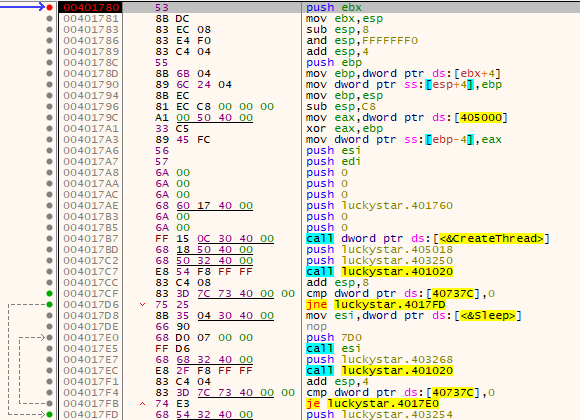Now we are there! It seems it creates a thread and waits until the thread quits. The program of this thread is located at 0x00401760 and luckily it's very small.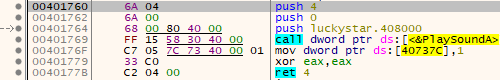The thread just plays the song and it doesn't seem to be important. After the song ends, it xors a region of data with the values gained by rand(). The region is called after we give an input string. However, the xored region is broken and the process dies. So, I run the program normally and attached during the input phase. I'm not sure why it fails with debugger, but I always get the correct code in this way. (Perhaps the debugger detection makes it complex.)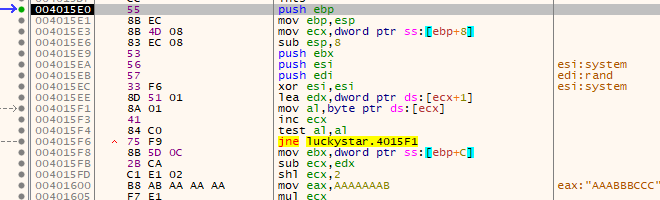As I analysed the piece of code, I found it is a base64 encoder. However, the uppercase of encoded string becomes lowercase and vice versa. It xors the base64 encoded string with rand() and compares to the valid one. This means we can find the valid base64 encoded string by xoring the xored data with rand() values. I got the sequence of rand() values by xoring my base64 string and the xored base64 string. The following code finds the valid base64 encoded string. sample = 'y\xf4_\xef1|$v\xa1\x87\xfe\x04B\xa3\xaf\xd0\xb0\xe0\xd0|\xb6Xz\x8d\xa3\xc79\x04\xfd\xcc\x97Ze}\xe9\xac\x98\nh8'
key = 'I\xe6W\xbd:G\x11L\x95\xbc\xee2r\xa0\xf0\xde\xac\xf2\x83V\x83In\xa9\xa6\xc5g<\xca\xc8\xcc\x05'
target = 'qufbqufbqufbqufbqufbqufbqufbqufbqufbqufbque=\n'
output = ""
i = 0
for (a, b) in zip(sample, target):
p = ord(a) ^ ord(b)
output += chr(p ^ ord(key[i]))
i += 1
if i == 0x20:
break
print(output)


The output is Agn0zNSXENvTAv9lmg5HDdrFtw8ZFq==. Notice that the uppercase becomes lowercase, and lower to upper, which means the real base64 value is aGN0ZnsxenVtaV9LMG5hdDRfTW8zfQ==. I decoded the base64 string and found the flag hctf{1zumi_K0nat4_Mo3}, haha.